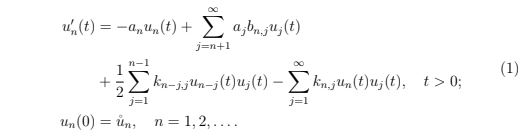# Dr Lyndsay Kerr

Project Title: Discrete Coagulation-Fragmentation Systems
There are many situations in nature and industrial processes where clusters of particles can merge together to form larger clusters and can break apart to form smaller clusters. For example, these processes occur in blood clotting, polymer science, aerosols and astrophysics (e.g. star formation from dust). We assume that each cluster is made up of identical units, each of which has mass equal to one, and we let un(t)  denote the density of clusters of size n at time t. Under suitable assumptions the evolution of clusters can then be described by an in finite set of ordinary differential equations of the form:For each n ∈ N, (1) describes how the density of clusters of size n changes with time. The coeficient an is the rate at which clusters of size n fragment, bn,j is the rate at which clusters of size n are produced when a larger cluster of size j fragments, kn,j is the rate at which clusters of size n merge with those of size j and ˚un is the initial density of clusters of size n. Recent research has focussed on autonomous equations, where the rates at which clusters fragment and coalesce are independent of time. Operator semigroups have been widely used in this research and much has been determined regarding the existence, uniqueness and various properties of solutions. In this project I aim to examine non-autonomous equations, where the rates an, bn,j and kn,j may depend on time. These time-dependent equations are important in connection to the powder production industry. However, not a great deal is currently known about these equations and there are many open questions that arise in relation to the non-autonomous system, such as: when does a solution exist? When is there a unique solution? What properties does this solution have? The aims of my project include answering these questions.Awarded: Carnegie PhD Scholarship

Field: Mathematics and Statistics

University: University of Strathclyde